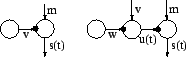Next: Linear Independent Factor Analysis Up: Example structures Previous: Example structures

## Variance Neurons

In most currently used models, the means of Gaussian nodes have hierarchical or dynamical models. In many real cases the variance is not constant either but it is more difficult to model it. We propose a variance neuron shown in Figure 2. It can convert a prediction of mean into a prediction of variance and thus allows to build hierarchical or dynamical models for the variance. In general the variance neuron results in a heavy-tailed super-Gaussian model for the Gaussian node it is attached to. This can be useful for instance in modelling outliers in the observations.Harri Valpola 2001-10-01